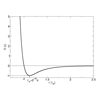#### You may also like### Integration Matcher

Can you match the charts of these functions to the charts of their integrals?### Brimful

Can you find the volumes of the mathematical vessels?### Lennard Jones Potential

Investigate why the Lennard-Jones potential gives a good approximate explanation for the behaviour of atoms at close ranges

# Interpolating Polynomials

##### Age 16 to 18 Challenge Level:

To find four points that a quadratic couldn't possibly fit, remember that quadratics only have one turning point.

To find the quadratic that fits three points, make sure you understand how you can add and subtract graphs, and what happens to the result. Don't try to fit all three points at once – fit two and then “fix” your line to fit the third.

Uniqueness: The Factor Theorem states that if $p$ is a polynomial and $p(a) = 0$, then there is a polynomial $q$ such that $p(x) = (x-a)q(x)$. What does this mean about the degrees of $p$ and $q$?

Finally, to prove two polynomials are equal, try proving their difference is zero.